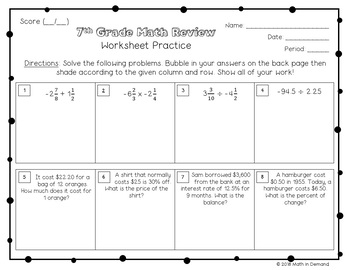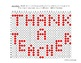# 7th Grade Math End of Year Review Hidden Message ActivitySubject
Resource Type
File Type

PDF

Also included in:
1. This is a growing bundle for my math worksheets that include a hidden bubble message. Currently there are 10 bubble in worksheets but there will be at least 20 ranging from 6th-9th grade math concepts.
\$34.50
\$31.05
Save \$3.45
Product Description
This is a short review on some 7th grade math concepts. There are 16 problems:

(2) Multiplying Fractions
(3) Dividing Fractions
(4) Dividing Decimals
(5) Calculating Unit Rate
(6) Discount (Markdown)
(7) Simple Interest and Balance
(8) Percent of Change
(9) Two-Step Equation
(10) Two-Step Equation involving a Fraction
(11) Two-Step Inequality
(12) Scale Factor
(13) Missing Angle in a Triangle
(14) Complementary Angle
(15) Calculate the Mean
(16) Probability

Students will solve these 16 problems then look at the answer code to determine which bubbles to shade in. This resource is great because the answer code makes it easy to self assess. Students can use this resource individually or in small groups.
Total Pages
Included
Teaching Duration
N/A
Report this Resource to TpT
Reported resources will be reviewed by our team. Report this resource to let us know if this resource violates TpT’s content guidelines.